# Drawing from very large databases

Drawing random samples from a very large database may be inefficient when done naively. We will explore in this article how to achieve this and study the algorithmic complexity of the proposed methods.

Introduction

Let suppose that we have a very large dataset D implemented in a relational database. Of course it may also be implemented in a NoSQL database but we will focus on a relational implementation because it will lead us to interesting reusable implementation concepts.

We will use PostgreSQL as a databse system with the Python language to illustrate our points. The methods we will see can be applied to other systems, even some NoSQL classical architectures (MongoDB with Node.js for example).

Now let suppose that each element u of the dataset has a probability P(u). We want to be able to draw one element from the dataset following the probability P. Let's also say that the dataset can be ordered ; this is not a loss of generality as in database systems rows can be ordered according to their row identifier or primary key.

The CDF (Cumulative Distribution Function) of probability P is given by :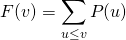We use in this definition the row order we have mentioned above. To draw from the dataset D a row according to probability P, it can be shown that the following method works :

• Draw a value x from a uniform distribution on [0, 1]
• Find v such as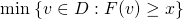i.e. the smallest v such as F(v) ≥ x. This is called the generalized inverse distribution function and is noted F-1(x).

Such a method works but how to implement it efficiently ? Searching a minimum over a set of N data is O(N) ; for large databases it would be too expensive.

We will investigate several methods to do this. First we will assume that P is uniform probabilities ; this is very often the case. In a second step we will generalize to arbitrary probabilities.

Database sequence

We said in the previous section that the dataset must be ordered, we can use for this purpose a unique key built using a database sequence. A sequence is generated by the database system and guaranties unique ascending numbers, generally incremented by 1. We can use this property to efficiently compute the F-1 for uniform probabilities.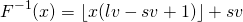Where lv and sv are respectively the last value and the start value of the sequence. Let's try this.

Create a sequence and a table that contains some strings to represent data (we don't mind about data here) and a column populated with a sequence :

When the data is populated we can check the sequence status :

We can use this to compute F-1 from a random number :

Then we can draw random data from the dataset1 table :

This is fast because the query use the index implicitly built by the unique constraint (this can be checked in the query execution plan, cf. the documentation). For comparison it is worth to try the following query that achieves the same result with no extra column (it is notably slower) :

Now we will do something similar this with Python. For optimization purpose, prepared statements may be used but this is beyond the scope of this article :

The program first accesses the sequence metadata in the database dictionary which costs O(1). Second it draws a random uniform number in [0, 1] and apply the inverse distribution function. Last it accesses the dataset using the database index which costs O(log N). Now let's run it :

Row suppression

The preceding example does not work anymore if there exists some holes in the sequence. Holes may appear in case of failed transaction at insertion time or later removal.

We can use acceptation / reject :

• Uniformly draw a row identifier between sv and lv.
• If a row exists with this identifier : return the data contained in the row (acceptation)
• If no row exists with this identifier : try again (reject)

It is proved that this leads to a uniform draw on the existing rows – mathematical proof is beyond the scope of this article.

Now we change the Python program as follow :

Note that if no row is found, None is returned.

And now, we try a draw until a row is found, (i.e. something else than None is returned) :

The program still accesses the database using the same index, multiple round trips may be necessary but it still costs O(log N).

Now let's create holes for test purposes, we randomly delete approximately half of the rows :

And now we run the Python code :

We see that in the run above we had to query the database 3 times to get an existing row (no luck – average is close to 2).

Cukoo filter

A Cuckoo filter is a structure that can assert that a given value does not belong to a set with certainty ; it allows deletion which make it preferable to a Bloom filter for our use case. See this paper for more information : Cuckoo Filter: Practically Better Than Bloom.

We will build such a filter in Python using this package : cuckoopy. Our filter will be fed with row identifiers and serialized to the database, if we obtain false when testing a given row identifier, we can reject this identifier with certainty.

First we create a table to save the Cuckoo filter :

The following Python program populates and serializes the filter :

It is a slow process but it should be done only once ; after initialization, the filter should me maintained in memory by the application (see bellow).

Now we modify the previous code in order to deserialize the filter from the database and compute the average round-trips number. Loading the filter is slow but it should be done only at application startup. Our goal here is to show that the number of round trips can be reduced, the following program shows average round trips with and without the Cukoo filter :

We observe that the number of average round-trips to the database fall close to the ideal value of 1.0 :

Of course serialization and deserialization of the Cuckoo filter is expensive. In a real life program it would be loaded only once and maintened in memory by inserting and deleting in the filter as well as in the database :

• for inserts, just before transaction commit
• for deletes, just after transaction commit

This way the filter is always coherent with the database. In real life again, the serialization and deserialization mechanisms will have to be far more clever than just the pickle way : snapshots of the filter can be regularly saved asynchronously but incremental updates will be needed to reload the filter, thus some logging is needed to track changes. The amount of work is worth only if a very high throughput is needed.

Non uniform probabilities

Let's go back to the general case. Now we assume that each row in the dataset has a weight. In the uniform case the weight was always 1 and the rid column can be seen as the cumulative weight in the row order (when no suppression occurs). We will use the same concept except than we will store a cumulative arbitrary weight.

The CDF of discrete probabilities is a step function and to each row of the dataset corresponds an interval [wi-1, wi] on which the CDF is constant. Thus inverting the CDF is the same as searching the row for which the given value is located in the corresponding interval.

Mathematically, we write that F is a step function this way :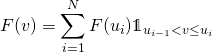Where N is the number of rows, u0 is a row before the first one. The generalized inverse can be written like this :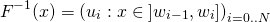Now let's see an example. First create and index a table with the weight bound columns as mentioned above :

The following Python code will insert 10 millions samples in the table :

The function insert_rows compute the cumulative weight lower and upper bounds for each row. The weights start from the highest weight in the database before insertion begins. Accessing the max is not very expensive : it will use the wupperix2 index and costs only O(log N).

Important note : the isolation level must be set to SERIALIZABLE ; at this level, transactions must behave as if they are executed serially. This means that if a table that was already accessed during the transaction is concurrently modified, the transaction fails. This ensure the consistency of the the weight bound values. In a real life program some retry mechanisms must be configured to guaranty that the data is finally inserted successfully. Generally speaking, such application must be carefully designed to ensure consistency with neither unnecessary locking nor time consuming control mechanisms.

Another note : for single inserts, SERIALIZABLE level is not mandatory but the insertion and weight computation must be done in a single query :
`INSERT INTO dataset2 (data, wlower, wupper)`
`SELECT <data>, MAX(wupper), MAX(wupper)+<weight>`
`FROM dataset2`
this should be used with caution : it will lead to performance issues for massive inserts done in loops.

When the program complete, the dataset look like this :

Now it is easy to draw a row according to the weights maintained in the table :

Important note : just after connection we have to send the command "SET enable_seqscan=OFF" ; this is mandatory for PostgreSQL because this RDBMS does not provide hints to fix bad explain plans and the optimizer is not good for estimating selectivity of criteria based on highly correlated columns. This directive forces the optimizer to always choose an Index Cond path. Other systems may not have this problem and / or different solutions.

This code is fast because it will use one of the two index :

1. Retrieving the highest weight uses wupperix2 and costs O(log N).
2. Retrieving the data uses also one of the index and costs O(log N) :
• wlowerix2 when the weight is low
• wupperix2 when the weight is high

Execute it a couple of times :

It is possible to delete rows and use acceptation / reject method but Cuckoo filters are not applicable for arbitrary probabilities.

It is not possible to modify the weight of a given line easily in all cases. However a row can be deleted and insert at the end of the table with its new weight.

Conditional probabilities

Sometimes we want to randomly draw a data but under a certain condition that must be met. For the sake of simplicity let's assume that the data can be split in multiple non overlapping classes given an equivalence relation : C1, C2, ..., CL. We should be able to draw data that belong to a given subset of the classes. It is enough to be able to draw from a single class because we can use the total probabilities formula :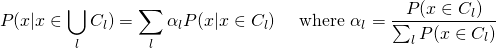This means that drawing from Ci ∪ Cj is equivalent to draw from Ci with probability αi or from Cj with probability αj.

A naive solution would be to implement a table for each class using the methods seen above and then proceed in two steps :

1. choose the table to draw with a probability proportional to its maximal cumulative weight,
2. draw from this table exactly the same way as described before.

But generally it is more appropriate to store all the data in the same table and add a column that contains the class identifier. To be able to draw from a single class, we only need to store the intervals needed for the marginal distribution for this class ; this means that the columns wlower and wupper are computed per class. Let's start our example by creating the proper structure and indexes :

This Python code shows how to insert data into the table ; current weights for each class are kept in a dictionary. As before, the consistency of the data is guarantied by the SERIALIZABLE level :

The query used to populate the weight dictionary is a little complicated, due to bad execution plan chosen by the optimizer when using a group by query (other database systems may not have this problem) – this one costs O(log N). The content of the table looks like this :

Now let's draw a data randomly that belongs to class C1 :

To draw a row that belongs to a subset of more than one class, the class must be first drawn according to weights in the dict returned by get_weights_upper. This can be done with numpy choice function. The cost is O(log N) because for all operations the indexes are used, as in the non-uniform non-conditional case seen above.

All Posts
×

Almost done…

We just sent you an email. Please click the link in the email to confirm your subscription!